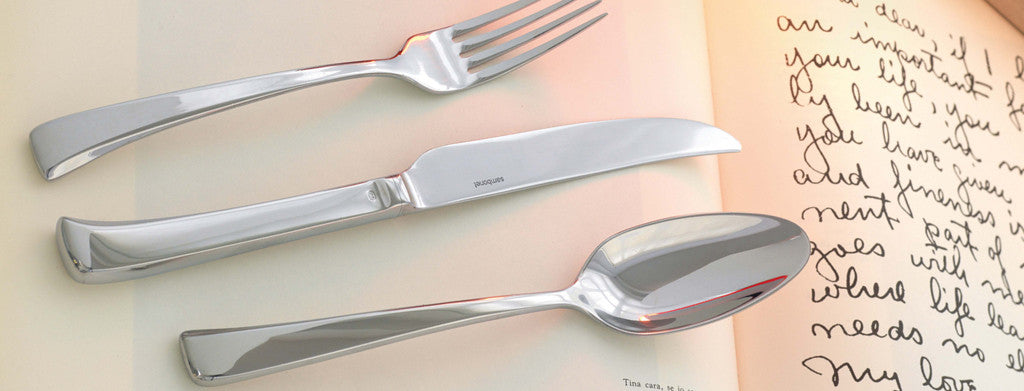Shopping Cart

### Refine

View all

#### Price# Cutlery£3.95 each (ex VAT)£3.29 each (ex VAT)£3.98 each (ex VAT)£1.71 each (ex VAT)£3.40 each (ex VAT)£5.48 each (ex VAT)£6.15 each (ex VAT)£12.24£0.88 each (ex VAT)£9.48 each (ex VAT)£0.55 each (ex VAT)£0.52 each (ex VAT)£9.48 each (ex VAT)£4.30 each (ex VAT)£4.83 each (ex VAT)£4.83 each (ex VAT)£6.15 each (ex VAT)£0.55 each (ex VAT)£0.53 each (ex VAT)£0.34 each (ex VAT)£0.46 each (ex VAT)£1.43 each (ex VAT)£1.21 each (ex VAT)£0.83 each (ex VAT)£1.43 each (ex VAT)£0.86 each (ex VAT)£0.76 each (ex VAT)£0.78 each (ex VAT)£0.59 each (ex VAT)£5.95 each (ex VAT)£4.71 each (ex VAT)£3.53 each (ex VAT)£4.75 each (ex VAT)£4.56 each (ex VAT)£4.23 each (ex VAT)£4.75 each (ex VAT)£9.31 each (ex VAT)£4.75 each (ex VAT)£4.30 each (ex VAT)£3.95 each (ex VAT)£9.48 each (ex VAT)£4.30 each (ex VAT)£3.40 each (ex VAT)£3.53 each (ex VAT)£4.30 each (ex VAT)£3.53 each (ex VAT)£4.14 each (ex VAT)£9.48 each (ex VAT)